# Main dynamics equations

Dynamics is the branch of physics that studies the causes of motion. We have gathered for you the main equations studied in this area of ​​knowledge.

Mechanics is the branch of Physics that studies movements and is subdivided into two parts: Dynamics and Kinematics . Dynamics is responsible for the study of the causes of movements. In dynamics, the study of Newton’s Laws and mechanical energy stands out . Kinematics can be understood as a mathematical analysis of movements.

Here are the main dynamics equations:

• Newton’s second law:

→ Newton’s second law says that the resultant force is the product of the mass and the acceleration of a body.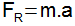→ The weight force is determined from Newton’s second law. Weight is the result of the product of the mass of a body and the acceleration due to gravity.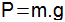• Frictional force

The friction force arises as a result of the contact between two surfaces and, in most cases, opposes the movement of an object. Its determination is made by the product of the normal force by the so-called coefficient of friction ( μ ) , a dimensionless quantity that characterizes surfaces, indicating whether they offer much or little resistance to movement.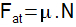• Hooke’s Law

The so-called Hooke’s law determines the elastic force (F EL ), a type of force that arises in the deformation of an elastic material . The determination of the elastic force is made through the product of the elastic constant of the material (K) by the deformation suffered by the material (X).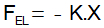The spring constant indicates whether the material is easily deformed or not. The negative sign arises from the fact that the elastic force always tries to bring the elastic material to the relaxed position, thus opposing the force that deforms it, so it is a type of restoring force.

• Centripetal force

Centripetal force pulls bodies on circular paths towards the center of the path.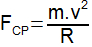For this equation, we can write:

CP = Centripetal force;

m = Mass of the body executing the circular path;

R = Radius of the circular path.

• work of a force

Work is the energy expended in performing an activity This quantity is the result of the product of force and displacement (considering the parallel force and displacement vectors).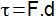• Energy

→ Kinetic energy: energy associated with velocity.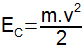For this equation, we can write:

C = Kinetic energy;

m = Mass;

v = Speed.

→ Gravitational potential energy : energy associated with the position of a body.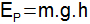For this equation, we can write:

m = Mass;

g = Gravity;

h = Height.

→ Mechanical energy : sum of potential and gravitational energies.

Check Also
Close